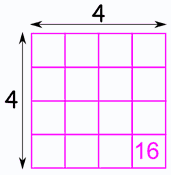Definition of

# Square (Numbers)To square a number: just multiply it by itself.

Example: "4 squared" is 4 × 4 = 16

Often shown with a little 2 in the corner like this: 42 = 16
that is said "4 squared equals 16"

A square number is the number we get after multiplying an integer (not a fraction) by itself.